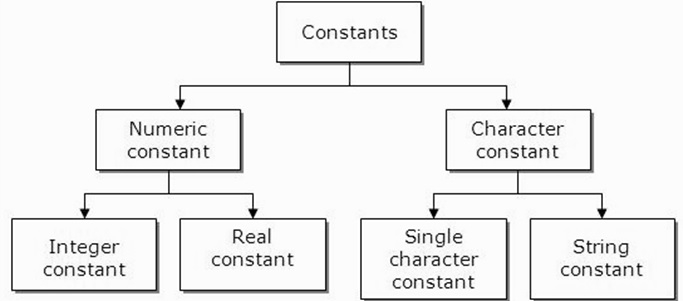# What are the constants with an example in C language?

Constant is also known as variable where once defined, the value never changes during the program execution. Thus, we can declare a variable as constant that refers to fixed values. It is also called as literals. Const keyword has to be used to define a constant.

## Syntax

The syntax for constant that is used in C programming language is given below −

const type VariableName;
(or)
const type *VariableName;

## Different types of constants

The different types of constants that are used in C programming language are as follows −

• Integer constants − For example: 1,0,34,4567

• Floating-point constants − For example: 0.0, 156.89, 23.456

• Octal & Hexadecimal constants − For example: Hexadecimal: 0x2a, 0xaa .. and Octal: 033, 024,..

• Character constants − For example: ‘a’, ‘B’, ‘x’

• String constants − For example: “TutorialsPoint”

The types of constants are also What ised in the diagram below −## Example 1

Following is the C program for determining the value of a number

Live Demo

#include<stdio.h>
int main(){
const int number=45;
int value;
int data;
printf("enter the data:");
scanf("%d",&data);
value=number*data;
printf("The value is: %d",value);
return 0;
}

## Output

When the above program is executed, it produces the following result −

enter the data:20
The value of number is: 900

In the above program, if we try to change the value of a number which is declared as constant, it displays an error.

## Example 2

Given below is the C program which gives an error, if we try to change the const value.

#include<stdio.h>
int main(){
const int number=45;
int data;
printf("enter the data:");
scanf("%d",&data);
number=number*data;
printf("The value of number is: %d",number);
return 0;
}

## Output

When the above program is executed, it produces the following result −

error